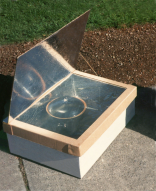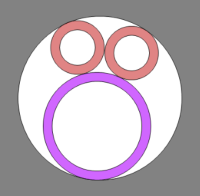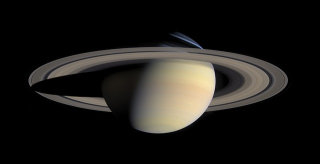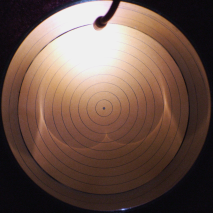# Geometry Expressions

## Mathematical Modeling

Geometry Expressions is a great mathematical modeling tool because it lets you express problems geometrically and extract algebra from the model.

You can then solve the algebraic model and explore the implications of your solution visually.

Alternatively, you can copy and paste the algebra into a CAS for solution, and paste the result back into Geometry Expressions.

We explore these different approaches in the models below.### Solar Cooker

A simple solar cooker consists of a box with a reflective lid.

If the box is positioned horizontally, and the sun is at angle φ to the horizontal, what angle should the lid be opened to?### Solar Concentration Ratio

We ask the question: What is the most sunlight a box like this can capture, and what angle would the lid be at this best case?### Pipe Fitting

Three pipes of different radii need to fit through a circular hole.

What is the radius of the smallest hole they'll fit through?### Regiomontanus' Problem

Thinking about the best place on earth to observe the rings of Saturn, Regiomontanus posed this problem:

Where on earth does a vertically suspended bar appear to be biggest?### Trammel

Archimedes trammel is a simple device for drawing an ellipse. Another way to draw an ellipse uses a piece of string and two pins.

To match the ellipse drawn by a particular trammel, how long should the string be, and where should the pins go?### Caustic

We model the curve formed by light reflecting in the walls of a cylinder.

We assume a point light source somewhere inside the cylinder and ask the question, where does the cusp in the caustic lie?